# Latest word problems

#### Number of problems found: 801

• The recommendedThe recommended price of the novel "Laughing Sun" is 285 SKK. The bookseller bought 60 pieces of the novel at the wholesale store and paid 82% of the recommended price (18% is his profit for selling books). For the recommended price, he sold 55 pieces ofThe regular quadrilateral pyramid has a base edge a = 1.56 dm and a height h = 2.05 dm. Calculate: a) the deviation angle of the sidewall plane from the base plane b) deviation angle of the side edge from the plane of the base
• Wooden bowls20 wooden bowls in the shape of a truncated cone should be painted on the outside and inside with wood varnish. We need 0.1 l of paint to paint 200 cm2. How many liters of paint do we have to buy if the bowls are 25 cm high, the bottom of the bowl has a d
• From plasticine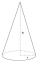Michael modeled from plasticine a 15 cm high pyramid with a rectangular base with the sides of the base a = 12 cm and b = 8 cm. From this pyramid, Janka modeled a rotating cone with a base diameter d = 10 cm. How tall was Janka's cone?
• Two vases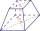Michaela has two vases in her collection. The first vase has the shape of a cone with a base diameter d = 20 cm; the second vase has the shape of a truncated cone with the lower base d1 = 25 cm and with the diameter of the upper base d2 = 15 cm. Which vas
• DecibelBy what percentage does the sound intensity increase if the sound intensity level increases by 1 dB?
• Cuboid diagonalsThe cuboid has dimensions of 15, 20 and 40 cm. Calculate its volume and surface, the length of the body diagonal and the lengths of all three wall diagonals.
• AquariumHow many liters of water can fit in an aquarium measuring 30, 15 and 20 cm?
• This year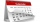This year 2020 is a leap year. How many leap years there had been be since 1904?
• Missing termWhat is the missing term in the sequence? _____, 13, 30, and 47?
• Find d 2Find d in an A. P. whose 5th term is 18 and 39th term is 120.
• In the 8In the A. P. 36, 39, 42, …, which term is 276?
• What is 9What is the 15th term; x1=1.5, d=4.5?
• 10th termWhat is the 10th term of the Arithmetic Progression if x1=4 and d=5?
• Find theFind the surface area of a regular quadrilateral pyramid which has a volume of 24 dm3 and a height of 45 cm.
• Six paintersSix painters paint an area of 120 m2 in 4 hours. How long does it take for 12 painters to paint 480 m2 with the same performance?
• Rhombus diagonalsIn the rhombus ABCD are given the sizes of diagonals e = 24 cm; f = 10 cm. Calculate the side length of the diamond and the size of the angles, calculate the content of the diamond
• Train tons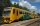The entire loaded train weighs 760 tons. It has a total of 30 wagons, each carrying 12 tons of grain and empty weighs 10 tons. What is the weight of the locomotive?After the history test, Michaella discovered that the ratio of her correct and incorrect answers is 5: 3. How many correct answers did Michaella have in the test, if she had 6 incorrect answers?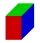Find the surface of the cuboid if its edges have the dimensions a, 2/3a, 2a